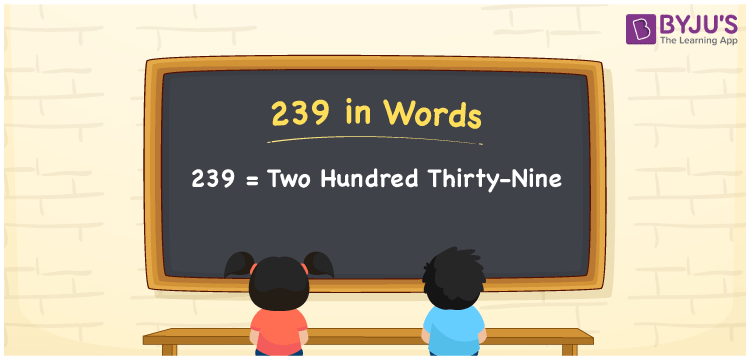# 239 in Words

The number 239 in words is expressed as “two hundred thirty-nine”. The numeral 239 is a natural number. 239 has three-digits and it lies between 238 and 240. In this article, let us have a look at the procedure of writing the number 239 in words using the place value system in detail.

 239 in Words: Two Hundred Thirty-nine. Two Hundred Thirty-nine in Numerical Form: 239.

## 239 in English Words## How to Write 239 in Words?

The place value table for the numeral 239 is given below.

 Hundreds Tens Ones 2 3 9

The expanded form of 239 is as follows:

= 2 × Hundred + 3 × Ten + 9 × One

= 2 × 100 + 3 × 10 + 9 × 1

= 200 + 30 + 9

= 239

= Two hundred thirty-nine

Hence, 239 in words is two hundred thirty-nine.

239 in words – Two hundred thirty-nine

Is 239 an odd number? – Yes

Is 239 an even number? – No

Is 239 a perfect square number? – No

Is 239 a perfect cube number? – No

Is 239 a prime number? – Yes

Is 239 a composite number? – No

## Frequently Asked Questions on 239 in Words

Q1

### Write 239 in words.

239 in words is two hundred thirty-nine.

Q2

### Simplify 200 + 39, and express it in words.

Simplifying 200 + 39, we get 239. Hence, 239 in words is two hundred thirty-nine.

Q3

### Is 239 a composite number?

No, 239 is not a composite number.